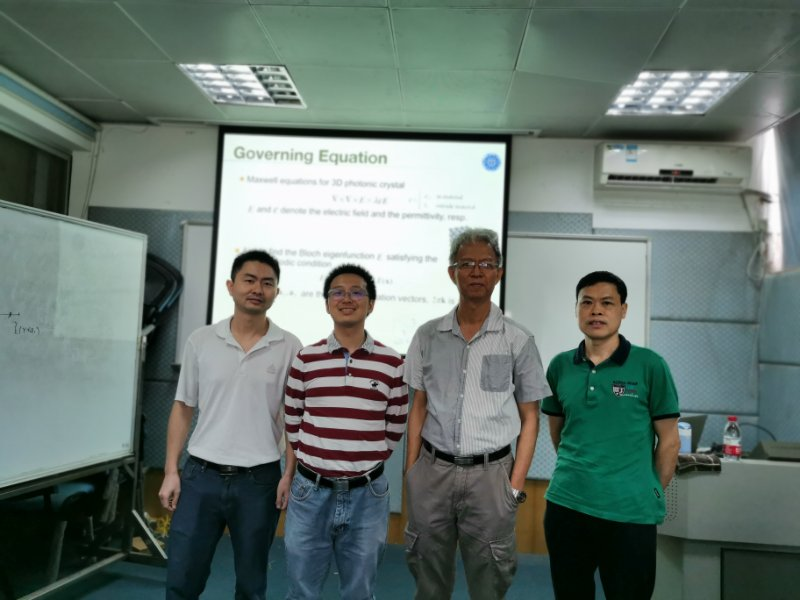Colloquiums and Conferences

# High-Performance Computing For Three Dimensional Maxwell’s Equation Arising From Photonic Crystals

Time: 2019-04-26 09:00:00

Topic: High-Performance Computing For Three Dimensional Maxwell’s Equation Arising From Photonic Crystals

Speaker: Prof Huang Tsung-ming      National Taiwan Normal University

Time: 2019-04-25 10:30--11:30

Location: Room 401 in School of Mathematical Sciences

Introduction:

The numerical simulation of the band structure of three-dimensional photonic crystals leads to large-scale generalized eigenvalue problems (GEPs). Due to a high dimensional subspace associated with the eigenvalue zeros, it is very challenging to solve the GEP. In this talk, we focus on proposing a highperformance computing method to solve GEP for all fourteen Bravais lattices. For each lattice, we derive the explicit matrix form of the discrete double-curl operator by using Yee’s scheme and classify all the matrices into four general types. The eigen-decompositions of these four general matrices are then derived. Based on these eigen-decompositions, the nullspace-free method is applied to exclude the zero eigenvalues from the associated generalized eigenvalue problem. Applying these theoretical results, a high-performance computing package FAME (Fast Algorithm for Maxwell’s Equations) with GPU acceleration is proposed to find the target eigenpairs.